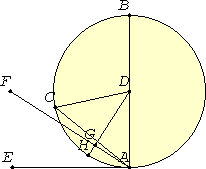# Proposition 16

The straight line drawn at right angles to the diameter of a circle from its end will fall outside the circle, and into the space between the straight line and the circumference another straight line cannot be interposed, further the angle of the semicircle is greater, and the remaining angle less, than any acute rectilinear angle.

Let ABC be a circle about D as center and AB as diameter.

I say that the straight line drawn from A at right angles to AB from its end will fall outside the circle.

For suppose it does not, but, if possible, let it fall within as CA, and join DC.I.5

Since DA equals DC, the angle DAC also equals the angle ACD.

I.17

But the angle DAC is right, therefore the angle ACD is also right. Thus, in the triangle ACD, the two angles DAC and ACD equal two right angles, which is impossible.

Therefore the straight line drawn from the point A at right angles to BA will not fall within the circle.

Similarly we can prove that neither will it fall on the circumference, therefore it will fall outside. Let it fall as AE.

I say next that into the space between the straight line AE and the circumference CHA another straight line cannot be interposed.

I.12

For, if possible, let another straight line be so interposed, as FA. Draw DG from the point D perpendicular to FA.

Then, since the angle AGD is right, and the angle DAG is less than a right angle, AD is greater than DG.

But DA equals DH, therefore DH is greater than DG, the less greater than the greater, which is impossible.

Therefore another straight line cannot be interposed into the space between the straight line and the circumference.

I say further that the angle of the semicircle contained by the straight line BA and the circumference CHA is greater than any acute rectilinear angle, and the remaining angle contained by the circumference CHA and the straight line AE is less than any acute rectilinear angle.

For, if there is any rectilinear angle greater than the angle contained by the straight line BA and the circumference CHA, and any rectilinear angle less than the angle contained by the circumference CHA and the straight line AE, then into the space between the circumference and the straight line AE a straight line will be interposed such as will make an angle contained by straight lines which is greater than the angle contained by the straight line BA and the circumference CHA, and another angle contained by straight lines which is less than the angle contained by the circumference CHA and the straight line AE.

Above

But such a straight line cannot be interposed, therefore there will not be any acute angle contained by straight lines which is greater than the angle contained by the straight line BA and the circumference CHA, nor yet any acute angle contained by straight lines which is less than the angle contained by the circumference CHA and the straight line AE.

Therefore the straight line drawn at right angles to the diameter of a circle from its end will fall outside the circle, and into the space between the straight line and the circumference another straight line cannot be interposed, further the angle of the semicircle is greater, and the remaining angle less, than any acute rectilinear angle.

Q.E.D.

# Corollary

From this it is clear that the straight line drawn at right angles to the diameter of a circle from its end touches the circle.

## Guide

#### Horn angles

Back in Book I, I.Def.8, Euclid defined an angle and included the possibility that the sides were not straight lines. Those whose sides were straight were called rectilinear angles in I.Def.9. Until this proposition we only saw rectilinear angles. In this proposition Euclid showed that the angle contained by the circumference CHA and the tangent straight line AE is less than any acute rectilinear angle. An angle of that sort is commonly called a horn angle. Its complement, the angle contained by the diameter and the circumference, is less than a right angle but greater than any acute rectilinear angle.

There’s a lot that could be said about these curvilinear angles, but their only appearance in the Elements is in this proposition.

Horn angles are infinitesimal with respect to rectilinear angles, that is, no multiple of a horn angle is greater than any rectilinear angle, or equivalently, no part (meaning fraction) of a rectilinear angle is less than a horn angle. The contemplation of horn angles leads to difficulties in the theory of proportions that’s developed in Book V.

#### Use of Proposition 16 and its corollary

This proposition is used in the proof of proposition IV.4 and two others in Book IV. The corollary is used in III.17, III.33, III.37, a few propositions in Book IV, and XII.16.